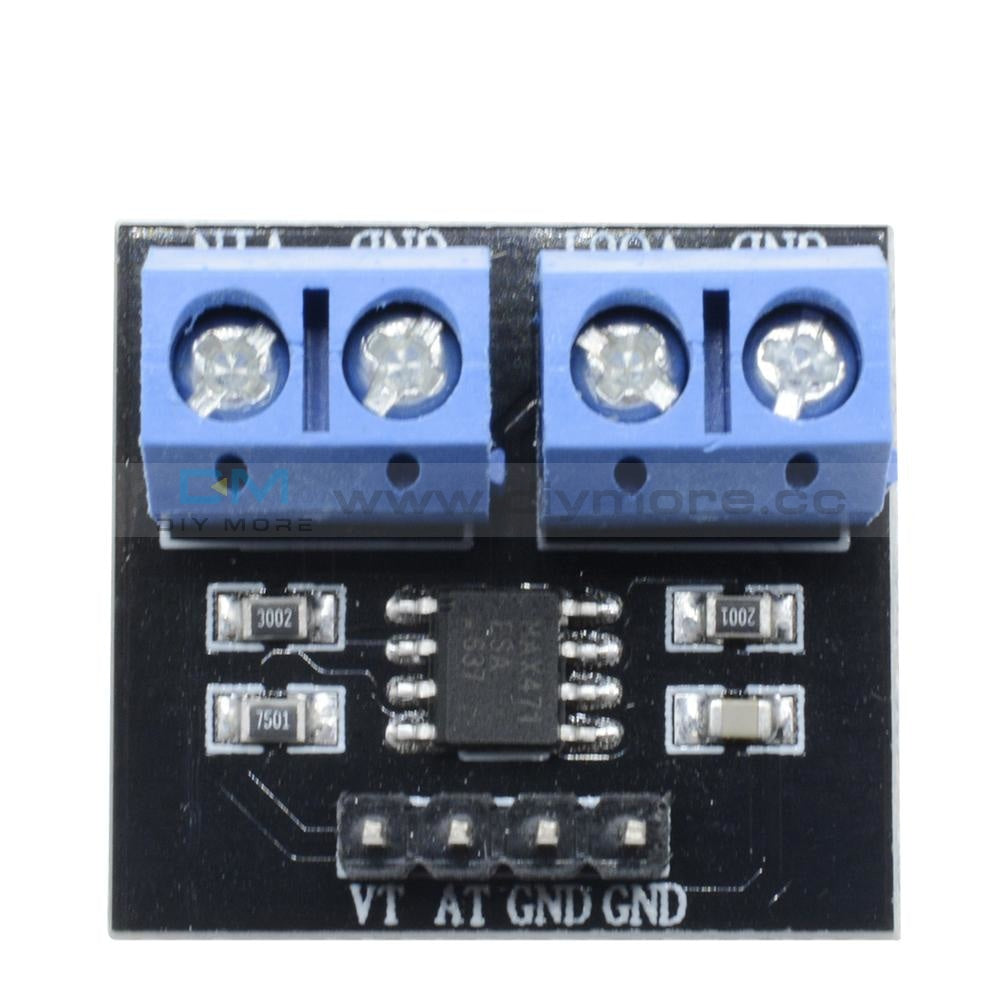### MAX471 Voltage Current Sensor Voltage Sensor Current Sensor for Arduino### MAX471 Voltage Current Sensor Voltage Sensor Current Sensor for Arduino

SKU:010819
Regular price \$4.99Features:
• This module can be used to test the current and voltage
• The principle of voltage test based on principle of resistive voltage divider design, can make the red terminal connector input voltage to 5 times smaller
• Arduino analog input voltages up to 5 v, the voltage detection module input voltage not greater than 5Vx5=25V (if using 3.3V systems, input voltage not greater than 3.3Vx5=16.5V)
• Arduino AVR chips have 10-bit AD, so this module simulates a resolution of 0.00489V (5V/1023), so the minimum voltage of input voltage detection module is 0.00489Vx5=0.02445V
• The MAX471 chip on the board used to test the consume current of the load.

Parameters:
Test Voltage range: DC3 – 25V (Arduino work on 5V) or DC3 – 16.5V (Arduino work on 3.3V)
Test Current range: DC 0 –3A

Package Included:
1 x Max471 Voltage Current Sensor• guaranteeQuality checked
• Free return Within 60 days
• Consultancy86-0755-85201155
Features:
• This module can be used to test the current and voltage
• The principle of voltage test based on principle of resistive voltage divider design, can make the red terminal connector input voltage to 5 times smaller
• Arduino analog input voltages up to 5 v, the voltage detection module input voltage not greater than 5Vx5=25V (if using 3.3V systems, input voltage not greater than 3.3Vx5=16.5V)
• Arduino AVR chips have 10-bit AD, so this module simulates a resolution of 0.00489V (5V/1023), so the minimum voltage of input voltage detection module is 0.00489Vx5=0.02445V
• The MAX471 chip on the board used to test the consume current of the load.

Parameters:
Test Voltage range: DC3 – 25V (Arduino work on 5V) or DC3 – 16.5V (Arduino work on 3.3V)
Test Current range: DC 0 –3A

Package Included:
1 x Max471 Voltage Current Sensor